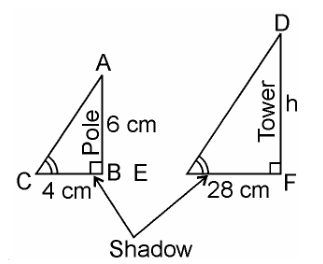Guru

# A vertical pole of a length 6 m casts a shadow 4m long on the ground and at the same time a tower casts a shadow 28 m long. Find the height of the tower. Q.15

• 0

I don’t know how to solve the problem of Triangles of exercise 6.3 of class 10th math, please help me to solve the problem of this question A vertical pole of a length 6 m casts a shadow 4m long on the ground and at the same time a tower casts a shadow 28 m long. Find the height of the tower.

Share

1. Given, Length of the vertical pole = 6m

Shadow of the pole = 4 m

Let Height of tower = h m

Length of shadow of the tower = 28 mIn ΔABC and ΔDEF,

∠C = ∠E (angular elevation of sum)

∠B = ∠F = 90°

∴ ΔABC ~ ΔDEF (AA similarity criterion)

∴ AB/DF = BC/EF (If two triangles are similar corresponding sides are proportional)

∴ 6/h = 4/28

⇒h = (6×28)/4

⇒ h = 6 × 7

⇒ = 42 m

Hence, the height of the tower is 42 m.

• 1